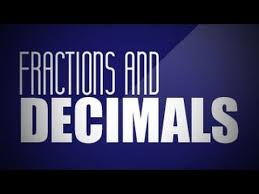# Fractions and Decimals: Ways to Represent Numbers

In this quiz, you will find questions based on the fractions and decimals and various operations performed on fractions and decimal numbers.Start Quiz

Find: 1.2 × 1.2

144 14.4 1.44 none of these

Find: 2.5 × 4

10 5 8 12

How much less is 10 km than 30.6 km?

10.6 km 20.6 km 30.6 km 10 km

The place value of 2 in 5.32 is

2 0.2 0.02 0.002

The place value of 2 in 2.38 is

1 2 20 0.2

Which of the following fraction?

7/4 19/4 14/5 4/11

1 – ( 1/5 ) is equal to

2/5

3/5

4/5

1/5

If 43m =0.086 then m has the value

0.002 0.02 2 0.2

0.01 × 0.01 = ______

0.0001 0.001 1 0.1

Find 0.2 x 0.3

0.6 0.06 6 None of these

Write the place value of 2 in the following decimal numbers : 2.56

5 .06 2 None of these

Find the area of rectangle whose length is 6.7 cm and breadth is 2 cm.

13 cm2 13.4 cm2 13.8 cm2 14 cm2

Which of the following is not an equivalent fraction of (3/5)?

(6/10)

(9/15)

(12/20)

(15/24)

2 + (1/4) is equal to

(7/4)

(9/4)

(5/4)

(11/4)

Apala ate (3/5) of an orange. The remaining orange was eaten by Meenu. What part of the orange was eaten by Meenu?

(1/5)

(2/5)

(3/5)

None of these

Quiz/Test Summary
Title: Fractions and Decimals: Ways to Represent Numbers
Questions: 15
Contributed by: